# 边界控制下耦合时空网络的固定时间同步Fixed-Time Synchronization of Coupled Spatio-Temporal Networks under Boundary Control

DOI: 10.12677/PM.2021.114067, PDF, HTML, XML, 下载: 19  浏览: 70

Abstract: In this paper, the fixed-time synchronization of coupled spatio-temporal network is concerned via boundary control. Firstly, a type of coupled spatio-temporal networks with mixed boundary con-ditions is introduced, in which the coupling term is composed of state coupling and spatial diffusion coupling. Secondly, by designing a boundary controller without a linear feedback term, some inequality techniques and Lyapunov theory are utilized, verifiable criteria are established to as-certain the fixed-time synchronization of coupled spatio-temporal networks. Finally, the effec-tiveness of the derived results is supported by an example with a numerical example.

1. 引言

2. 预备知识

2.1. 模型描述

$\left\{\begin{array}{l}\frac{\partial {z}_{r}\left(x,t\right)}{\partial t}=D\Delta {z}_{r}\left(x,t\right)+A{z}_{r}\left(x,t\right)+Bf\left({z}_{r}\left(x,t\right)\right)+\epsilon \underset{j=1}{\overset{m}{\sum }}{w}_{rj}\Gamma \Delta {z}_{j}\left(x,t\right)+\epsilon \underset{j=1}{\overset{m}{\sum }}{w}_{rj}\stackrel{^}{\Gamma }\Delta {z}_{j}\left(x,t\right),\\ {z}_{r}\left(x,0\right)={z}_{r}^{0}\left(x\right),\text{\hspace{0.17em}}\text{\hspace{0.17em}}x\in \left[0,L\right],\end{array}$ (1)

${z}_{r}\left(x,t\right)={0}_{n},\text{\hspace{0.17em}}\text{\hspace{0.17em}}{\frac{\partial {z}_{r}\left(x,t\right)}{\partial x}|}_{x=L}={U}_{r}\left(t\right),$ (2)

$\left\{\begin{array}{l}\frac{\partial \xi \left(x,t\right)}{\partial t}=D\Delta \xi \left(x,t\right)+A\xi \left(x,t\right)+Bf\left(\xi \left(x,t\right)\right),\\ \xi \left(x,0\right)={\xi }^{0}\left(x\right),\text{\hspace{0.17em}}\text{\hspace{0.17em}}x\in \left[0,L\right],\\ \xi \left(x,t\right)={\frac{\partial \xi \left(x,t\right)}{\partial x}|}_{x=L}={0}_{n},\text{\hspace{0.17em}}\text{\hspace{0.17em}}t\in \left[0,+\infty \right).\end{array}$ (3)

$\left\{\begin{array}{l}\frac{\partial {\sigma }_{r}\left(x,t\right)}{\partial t}=D\Delta {\sigma }_{r}\left(x,t\right)+A{\sigma }_{r}\left(x,t\right)+B\stackrel{˜}{f}\left({\sigma }_{r}\left(x,t\right)\right)+\epsilon \underset{j=1}{\overset{m}{\sum }}{w}_{rj}\Gamma \Delta {\sigma }_{j}\left(x,t\right)+\epsilon \underset{j=1}{\overset{m}{\sum }}{w}_{rj}\stackrel{^}{\Gamma }\Delta {\sigma }_{j}\left(x,t\right),\\ {\sigma }_{r}\left(x,0\right)={z}_{r}^{0}\left(x\right)-{\xi }^{0}\left(x\right),\text{\hspace{0.17em}}\text{\hspace{0.17em}}x\in \left[0,L\right],\\ {\sigma }_{r}\left(0,t\right)={0}_{n},\text{\hspace{0.17em}}\text{\hspace{0.17em}}{\frac{\partial {\sigma }_{r}\left(x,t\right)}{\partial x}|}_{x=L}={U}_{r}\left(t\right),\text{\hspace{0.17em}}\text{\hspace{0.17em}}t\in \left[0,+\infty \right),\end{array}$ (4)

$T\left(\sigma \left(x,0\right)\right)\le {T}_{\mathrm{max}}$，则称系统(1)是固定时间同步的。

2.2. 引理和假设

${\int }_{0}^{L}{F}^{\text{T}}\left(r\right)\begin{array}{c}QF\left(r\right)\text{d}r\end{array}\le \frac{4{L}^{2}}{{\pi }^{2}}{\int }_{0}^{L}{\left(\frac{\text{d}F\left(r\right)}{\text{d}r}\right)}^{\text{T}}Q\left(\frac{\text{d}F\left(r\right)}{\text{d}r}\right)\text{d}r.$

$\frac{\text{d}}{\text{d}t}F\left(x\left(t\right)\right)\le aF\left(x\left(t\right)\right)-b{F}^{\eta }\left(x\left(t\right)\right)-c{F}^{\delta }\left(x\left(t\right)\right),\text{\hspace{0.17em}}\text{\hspace{0.17em}}x\left(t\right)\in {R}^{n}\\left\{\begin{array}{c}{0}_{n}\end{array}\right\},$

(1) 若 $a\le \text{0}$，则当 $t\ge T\left(x\left(0\right)\right)$ 时有 $F\left(x\left(t\right)\right)=\text{0}$$x\left(t\right)=0$，且 $T\left(x\left(0\right)\right)$ 满足：

$T\left(x\left(0\right)\right)\le {T}_{\mathrm{max}}^{1}=\frac{\pi }{\delta -\eta }{\left(\frac{b}{c}\right)}^{v}\mathrm{csc}\left(v\pi \right),$

(2) 若 $0，则当 $t\ge T\left(x\left(0\right)\right)$ 时有 $F\left(x\left(t\right)\right)=\text{0}$$x\left(t\right)=0$，且 $T\left(x\left(0\right)\right)$ 满足：

$T\left(x\left(0\right)\right)\le {T}_{\mathrm{max}}^{2}=\frac{\pi \mathrm{csc}\left(v\pi \right)}{c\left(\delta -\eta \right)}{\left(\frac{c}{b-a}\right)}^{1-v}I\left(\frac{c}{b+c-a},v,1-v\right)+\frac{\pi \mathrm{csc}\left(v\pi \right)}{b\left(\delta -\eta \right)}{\left(\frac{b}{c-a}\right)}^{v}I\left(\frac{b}{b+c-a},1-v,v\right),$

$I\left(x,y,z\right)=\frac{1}{B\left(y,z\right)}{\int }_{0}^{x}{t}^{y-1}{\left(1-t\right)}^{z-1}\text{d}t,$

$B\left(y,z\right)={\int }_{0}^{1}{t}^{y-1}{\left(1-t\right)}^{z-1}\text{d}t,$

(1) 若 $0$\eta +\delta =\text{2}$，则当 $t\ge T\left(x\left(0\right)\right)$ 时有 $F\left(x\left(t\right)\right)=\text{0}$$x\left(t\right)=0$，且 $T\left(x\left(0\right)\right)$ 满足：

$T\left(x\left(0\right)\right)\le {T}_{\mathrm{max}}^{3}=\frac{1}{\delta -1}\frac{2}{\sqrt{4bc-{a}^{2}}}\left(\frac{\pi }{2}+\mathrm{arctan}\left(\frac{2}{\sqrt{4bc-{a}^{2}}}\right)\right).$

$\underset{k=1}{\overset{s}{\sum }}{c}_{k}^{u}\ge {\left(\underset{k=1}{\overset{s}{\sum }}{c}_{k}\right)}^{u},\text{\hspace{0.17em}}\text{\hspace{0.17em}}\underset{k=1}{\overset{s}{\sum }}{c}_{k}^{v}\ge {s}^{1-v}{\left(\underset{k=1}{\overset{s}{\sum }}{c}_{k}\right)}^{v}.$

$‖f\left(a\right)-f\left(b\right)‖\le \rho ‖a-b‖.$

3. 主要结果及证明

${U}_{r}\left(t\right)=\left\{\begin{array}{l}-\alpha \begin{array}{c}{\Psi }_{r}\left(L,t\right)\end{array}{V}_{r}^{p}\left(t\right)-\beta \begin{array}{c}{\Psi }_{r}\left(L,t\right)\end{array}{V}_{r}^{q}\left(t\right),\text{\hspace{0.17em}}\text{\hspace{0.17em}}{\sigma }_{r}\left(L,t\right)\ne {0}_{n},\\ {0}_{n},\text{\hspace{0.17em}}\text{\hspace{0.17em}}{\sigma }_{r}\left(L,t\right)={0}_{n},\text{​}\text{​}\text{​}\text{​}\text{​}\text{​}\text{​}\text{​}\end{array}$ (5)

${\Psi }_{r}\left(L,t\right)=\frac{{\sigma }_{r}\left(L,t\right)}{{‖{\sigma }_{r}\left(L,t\right)‖}^{2}},\text{\hspace{0.17em}}\text{\hspace{0.17em}}{V}_{r}\left(t\right)={\int }_{0}^{L}{\sigma }_{r}^{\text{T}}\left(x,t\right)\Theta {\sigma }_{r}\left(x,t\right)\text{d}x.$

$\sum ={I}_{m}\otimes \left({\left[\Theta A\right]}^{s}+\rho ‖{\left[\Theta B\right]}^{s}‖{I}_{n}\right)-\frac{{\pi }^{2}}{2{L}^{2}}\Pi +\epsilon {\left[W\otimes \Theta \stackrel{^}{\Gamma }\right]}^{s}.$

$\left\{\begin{array}{l}\frac{\partial \sigma \left(x,t\right)}{\partial t}=\left({I}_{m}\otimes D\right)\Delta \sigma \left(x,t\right)+\left({I}_{m}\otimes A\right)\sigma \left(x,t\right)+\left({I}_{m}\otimes B\right)F\left(\sigma \left(x,t\right)\right)\\ \text{}+\epsilon \left(W\otimes \Gamma \right)\Delta \sigma \left(x,t\right)+\epsilon \left(W\otimes \stackrel{^}{\Gamma }\right)\sigma \left(x,t\right),\text{\hspace{0.17em}}\text{\hspace{0.17em}}x\in \left(0,L\right),\\ \sigma \left(x,0\right)={z}^{0}\left(x\right)-{\xi }^{0}\left(x\right),\text{\hspace{0.17em}}\text{\hspace{0.17em}}x\in \left(0,L\right),\\ \sigma \left(0,t\right)={0}_{nm},\text{\hspace{0.17em}}\text{\hspace{0.17em}}{\frac{\partial \sigma \left(x,t\right)}{\partial x}|}_{x=L}=U\left(t\right),\text{}t\in \left[0,+\infty \right),\end{array}$ (6)

$\prod ={I}_{m}\otimes \Theta D+\epsilon W\otimes \Theta \Gamma >0,$

(1) 当 ${\lambda }_{\text{max}}\left(\stackrel{˜}{\sum }\right)\le \text{0}$ 时，受控网络(1)是固定时间同步的，且停息时间 ${T}^{*}$ 满足

${T}^{*}\le {\stackrel{¯}{T}}_{1}=\frac{\pi }{\stackrel{˜}{\alpha }\left(\delta -\eta \right)}{\left(\frac{\stackrel{˜}{\alpha }}{\stackrel{˜}{\beta }}\right)}^{v}\mathrm{csc}\left(v\pi \right),$

(2) 当 $0<{\lambda }_{\mathrm{max}}\left(\stackrel{˜}{\sum }\right)\begin{array}{c}<\end{array}\mathrm{min}\left\{\stackrel{˜}{\alpha },\stackrel{˜}{\beta }\right\}$ 时，受控网络(1)在固定时间 ${T}^{*}$ 内实现同步，且 ${T}^{*}$ 满足

$\begin{array}{l}{T}^{*}\le {\stackrel{¯}{T}}_{2}=\frac{\pi \mathrm{csc}\left(v\pi \right)}{\stackrel{˜}{\beta }\left(p-p\right)}{\left(\frac{\stackrel{˜}{\beta }}{\stackrel{˜}{\alpha }-{\lambda }_{\mathrm{max}}\left(\stackrel{˜}{\sum }\right)}\right)}^{1-v}I\left(\frac{\stackrel{˜}{\beta }}{\stackrel{˜}{\beta }+\stackrel{˜}{\alpha }-{\lambda }_{\mathrm{max}}\left(\stackrel{˜}{\sum }\right)},v,1-v\right)\\ \text{\hspace{0.17em}}\text{\hspace{0.17em}}\text{\hspace{0.17em}}\text{\hspace{0.17em}}\text{\hspace{0.17em}}\text{\hspace{0.17em}}\text{\hspace{0.17em}}\text{\hspace{0.17em}}\text{\hspace{0.17em}}\text{\hspace{0.17em}}\text{\hspace{0.17em}}\text{\hspace{0.17em}}\text{\hspace{0.17em}}+\frac{\pi \mathrm{csc}\left(v\pi \right)}{\stackrel{˜}{\alpha }\left(\stackrel{˜}{\beta }-{\lambda }_{\mathrm{max}}\left(\stackrel{˜}{\sum }\right)\right)}{\left(\frac{\stackrel{˜}{\alpha }}{\stackrel{˜}{\beta }-{\lambda }_{\mathrm{max}}\left(\stackrel{˜}{\sum }\right)}\right)}^{v}I\left(\frac{\stackrel{˜}{\alpha }}{\stackrel{˜}{\beta }+\stackrel{˜}{\alpha }-{\lambda }_{\mathrm{max}}\left(\stackrel{˜}{\sum }\right)},1-v,v\right).\end{array}$

(3) 当 $p+q=2$$0<{\lambda }_{\mathrm{max}}\left(\stackrel{˜}{\sum }\right)<2\sqrt{\stackrel{˜}{\alpha }\stackrel{˜}{\beta }}$ 时，受控网络(1)是固定时间同步的，且停息时间 ${T}^{*}$ 满足

${T}^{*}\le {\stackrel{¯}{T}}_{3}=\frac{1}{q-1}\frac{2}{\sqrt{4\stackrel{˜}{\alpha }\stackrel{˜}{\beta }-{\lambda }_{\mathrm{max}}^{2}\left(\stackrel{˜}{\sum }\right)}}\left(\frac{\pi }{2}+\mathrm{arctan}\left(\frac{{\lambda }_{\mathrm{max}}\left(\stackrel{˜}{\sum }\right)}{\sqrt{4\stackrel{˜}{\alpha }\stackrel{˜}{\beta }-{\lambda }_{\mathrm{max}}^{2}\left(\stackrel{˜}{\sum }\right)}}\right)\right).$

$V\left(t\right)={\int }_{0}^{L}{\sigma }^{\text{T}}\left(x,t\right)\left({I}_{m}\otimes \Theta \right)\sigma \left(x,t\right)\text{d}x.$

$\begin{array}{c}\stackrel{˙}{V}\left(t\right)=2{\int }_{0}^{L}\left[{\sigma }^{\text{T}}\left(x,t\right)\left({I}_{m}\otimes \Theta D\right)\Delta \sigma \left(x,t\right)\\ \text{\hspace{0.17em}}+\left({I}_{m}\otimes \Theta A\right)\sigma \left(x,t\right)+\left({I}_{m}\otimes \Theta B\right)F\left(\sigma \left(x,t\right)\right)\right]\text{d}x\\ \text{\hspace{0.17em}}+2\epsilon {\int }_{0}^{L}\left[{\sigma }^{\text{T}}\left(x,t\right)\left(W\otimes \Theta \Gamma \right)\Delta \sigma \left(x,t\right)\text{d}x\\ \text{\hspace{0.17em}}+2\epsilon {\int }_{0}^{L}\left[{\sigma }^{\text{T}}\left(x,t\right)\left(W\otimes \Theta \stackrel{^}{\Gamma }\right)\sigma \left(x,t\right)\text{d}x.\end{array}$ (7)

$\begin{array}{l}2{\int }_{0}^{L}{\sigma }^{\text{T}}\left(x,t\right)\left({I}_{m}\otimes \Theta B\right)F\left(\sigma \left(x,t\right)\right)\text{d}x\\ =\underset{r=1}{\overset{m}{\sum }}{\int }_{0}^{L}{\sigma }_{r}^{\text{T}}\left(x,t\right)\left(\Theta B+{B}^{\text{T}}\Theta \right)\stackrel{˜}{f}\left({\sigma }_{r}\left(x,t\right)\right)\text{d}x\\ \le \underset{r=1}{\overset{m}{\sum }}{\int }_{0}^{L}‖{\sigma }_{r}^{\text{T}}\left(x,t\right)‖×‖\left(\Theta B+{B}^{\text{T}}\Theta \right)‖×‖\stackrel{˜}{f}\left({\sigma }_{r}\left(x,t\right)\right)‖\text{d}x\\ \le {\int }_{0}^{L}{\sigma }^{\text{T}}\left(x,t\right)\left({I}_{m}\otimes \rho ‖{\left[\Theta B\right]}^{s}‖{I}_{n}\right)\sigma \left(x,t\right)\text{d}x.\end{array}$ (8)

$\begin{array}{l}2{\int }_{0}^{L}{\sigma }^{\text{T}}\left(x,t\right)\left({I}_{m}\otimes \Theta D\right)\Delta \sigma \left(x,t\right)\text{d}x\\ =2\underset{r=1}{\overset{m}{\sum }}{\int }_{0}^{L}{\sigma }_{r}^{\text{T}}\left(x,t\right)\Theta D\text{}{\frac{\partial {\sigma }_{r}\left(x,t\right)}{\partial x}|}_{0}^{L}\text{d}x-2\underset{r=1}{\overset{m}{\sum }}{\int }_{0}^{L}{\left(\frac{\partial {\sigma }_{r}\left(x,t\right)}{\partial x}\right)}^{\text{T}}\Theta D\left(\frac{\partial {\sigma }_{r}\left(x,t\right)}{\partial x}\right)dx\\ =2\underset{r=1}{\overset{m}{\sum }}{\sigma }_{r}^{\text{T}}\left(L,t\right)\Theta D\begin{array}{c}{U}_{r}\left(t\right)\end{array}-2\underset{r=1}{\overset{m}{\sum }}{\int }_{0}^{L}{\left(\frac{\partial {\sigma }_{r}\left(x,t\right)}{\partial x}\right)}^{\text{T}}\Theta D\left(\frac{\partial {\sigma }_{r}\left(x,t\right)}{\partial x}\right)\text{d}x\\ =2{\sigma }^{\text{T}}\left(L,t\right)\left({I}_{m}\otimes \Theta D\right)U\left(t\right)-2{\int }_{0}^{L}{\left(\frac{\partial {\sigma }_{r}\left(x,t\right)}{\partial x}\right)}^{\text{T}}\left({I}_{m}\otimes \Theta D\right)\left(\frac{\partial {\sigma }_{r}\left(x,t\right)}{\partial x}\right)\text{d}x.\end{array}$ (9)

$\begin{array}{l}2\epsilon {\int }_{0}^{L}{\sigma }^{\text{T}}\left(x,t\right)\left(W\otimes \Theta \Gamma \right)\Delta \sigma \left(x,t\right)\text{d}x\\ =2\epsilon \underset{r=1}{\overset{m}{\sum }}{\int }_{0}^{L}{\sigma }_{r}^{\text{T}}\left(x,t\right){w}_{rj}\Theta \Gamma {\frac{\partial {\sigma }_{r}\left(x,t\right)}{\partial x}|}_{0}^{L}\text{d}x\\ \text{\hspace{0.17em}}\text{\hspace{0.17em}}\text{ }-2\epsilon \underset{r=1}{\overset{m}{\sum }}\underset{j=1}{\overset{m}{\sum }}{\int }_{0}^{L}{\left(\frac{\partial {\sigma }_{r}\left(x,t\right)}{\partial x}\right)}^{\text{T}}{w}_{rj}\Theta \Gamma \left(\frac{\partial {\sigma }_{j}\left(x,t\right)}{\partial x}\right)\text{d}x\\ =2\epsilon {\sigma }^{\text{T}}\left(L,t\right)\left(W\otimes \Theta \Gamma \right)U\left(t\right)-2\epsilon {\int }_{0}^{L}{\left(\frac{\partial \sigma \left(x,t\right)}{\partial x}\right)}^{\text{T}}\left(W\otimes \Theta \Gamma \right)\left(\frac{\partial \sigma \left(x,t\right)}{\partial x}\right)\text{d}x.\end{array}$ (10)

$\begin{array}{l}-2{\int }_{0}^{L}{\left(\frac{\partial \sigma \left(x,t\right)}{\partial x}\right)}^{\text{T}}\left({I}_{m}\otimes \Theta D\right)\left(\frac{\partial \sigma \left(x,t\right)}{\partial x}\right)\text{d}x-2\epsilon {\int }_{0}^{L}{\left(\frac{\partial \sigma \left(x,t\right)}{\partial x}\right)}^{\text{T}}\left(W\otimes \Theta \Gamma \right)\left(\frac{\partial \sigma \left(x,t\right)}{\partial x}\right)\text{d}x\\ =-2{\int }_{0}^{L}{\left(\frac{\partial \sigma \left(x,t\right)}{\partial x}\right)}^{\text{T}}\prod \left(\frac{\partial \sigma \left(x,t\right)}{\partial x}\right)\text{d}x\le -\frac{{\pi }^{2}}{2{L}^{2}}{\int }_{0}^{L}{\sigma }^{\text{T}}\left(x,t\right)\begin{array}{c}\prod \end{array}\begin{array}{c}\sigma \left(x,t\right)\end{array}\text{d}x.\end{array}$ (11)

$\begin{array}{l}{\sigma }^{\text{T}}\left(L,t\right)\left({I}_{m}\otimes \Theta D\right)U\left(t\right)\\ =-\alpha \underset{r=1}{\overset{m}{\sum }}{\sigma }_{r}^{\text{T}}\left(L,t\right)\Theta D{\sigma }_{r}\left(L,t\right)\frac{{\left({V}_{r}\left(t\right)\right)}^{p}}{{‖{\sigma }_{r}\left(L,t\right)‖}^{2}}-\beta \underset{r=1}{\overset{m}{\sum }}{\sigma }_{r}^{\text{T}}\left(L,t\right)\Theta D{\sigma }_{r}\left(L,t\right)\frac{{\left({V}_{r}\left(t\right)\right)}^{q}}{{‖{\sigma }_{r}\left(L,t\right)‖}^{2}}\\ =-\alpha \underset{r=1}{\overset{m}{\sum }}\underset{k=1}{\overset{n}{\sum }}{\sigma }_{r}^{k}\left(L,t\right){\Theta }_{k}{d}_{k}{\sigma }_{r}^{k}\left(L,t\right)\frac{{\left({V}_{r}\left(t\right)\right)}^{p}}{{‖{\sigma }_{r}\left(L,t\right)‖}^{2}}-\beta \underset{r=1}{\overset{m}{\sum }}\underset{k=1}{\overset{n}{\sum }}{\sigma }_{r}^{k}\left(L,t\right){\Theta }_{k}{d}_{k}{\sigma }_{r}^{k}\left(L,t\right)\frac{{\left({V}_{r}\left(t\right)\right)}^{q}}{{‖{\sigma }_{r}\left(L,t\right)‖}^{2}}\\ =-\alpha \underset{k=1}{\overset{n}{\sum }}{\left({\sigma }^{k}\left(L,t\right)\right)}^{\text{T}}{\Theta }_{k}{d}_{k}{I}_{m}{\stackrel{˜}{\sigma }}^{k}\left(L,t\right)-\beta \underset{k=1}{\overset{n}{\sum }}{\left({\sigma }^{k}\left(L,t\right)\right)}^{\text{T}}{\Theta }_{k}{d}_{k}{I}_{m}{\stackrel{^}{\sigma }}^{k}\left(L,t\right)\end{array}$ (12)

$\begin{array}{l}\epsilon {\sigma }^{\text{T}}\left(L,t\right)\left(W\otimes \Theta \Gamma \right)U\left(t\right)\\ =-\epsilon \alpha \underset{r=1}{\overset{m}{\sum }}\underset{j=1}{\overset{m}{\sum }}{\sigma }_{r}^{\text{T}}\left(L,t\right){\omega }_{rj}\Theta \Gamma {\sigma }_{j}\left(L,t\right)\frac{{\left({V}_{j}\left(t\right)\right)}^{p}}{{‖{\sigma }_{j}\left(L,t\right)‖}^{2}}-\epsilon \beta \underset{r=1}{\overset{m}{\sum }}\underset{j=1}{\overset{m}{\sum }}{\sigma }_{r}^{\text{T}}\left(L,t\right){\omega }_{rj}\Theta \Gamma {\sigma }_{j}\left(L,t\right)\frac{{\left({V}_{j}\left(t\right)\right)}^{q}}{{‖{\sigma }_{j}\left(L,t\right)‖}^{2}}\\ =-\epsilon \alpha \underset{r=1}{\overset{m}{\sum }}\underset{j=1}{\overset{m}{\sum }}\underset{k=1}{\overset{n}{\sum }}{\sigma }_{r}^{k}\left(L,t\right){\omega }_{rj}{\Theta }_{k}{d}_{k}{\sigma }_{r}^{k}\left(L,t\right)\frac{{\left({V}_{j}\left(t\right)\right)}^{p}}{{‖{\sigma }_{j}\left(L,t\right)‖}^{2}}-\epsilon \beta \underset{r=1}{\overset{m}{\sum }}\underset{j=1}{\overset{m}{\sum }}\underset{k=1}{\overset{n}{\sum }}{\sigma }_{r}^{k}\left(L,t\right){\omega }_{rj}{\Theta }_{k}{d}_{k}{\sigma }_{r}^{k}\left(L,t\right)\frac{{\left({V}_{r}\left(t\right)\right)}^{q}}{{‖{\sigma }_{r}\left(L,t\right)‖}^{2}}\\ =-\epsilon \alpha \underset{k=1}{\overset{n}{\sum }}{\left({\sigma }^{k}\left(L,t\right)\right)}^{\text{T}}{\Theta }_{k}{\gamma }_{k}W{\stackrel{˜}{\sigma }}^{k}\left(L,t\right)-\epsilon \beta \underset{k=1}{\overset{n}{\sum }}{\left({\sigma }^{k}\left(L,t\right)\right)}^{\text{T}}{\Theta }_{k}{\gamma }_{k}W{\stackrel{^}{\sigma }}^{k}\left(L,t\right).\end{array}$ (13)

${\sigma }^{k}\left(x,t\right)={\left({\sigma }_{1}^{k}\left(x,t\right),{\sigma }_{2}^{k}\left(x,t\right),\cdots ,{\sigma }_{m}^{k}\left(x,t\right)\right)}^{\text{T}},$

$\begin{array}{l}{\stackrel{˜}{\sigma }}^{k}\left(x,t\right)={\left({\sigma }_{1}^{k}\left(x,t\right)\frac{{\left({V}_{1}\left(t\right)\right)}^{p}}{{‖{\sigma }_{1}\left(L,t\right)‖}^{2}},{\sigma }_{2}^{k}\left(x,t\right)\frac{{\left({V}_{2}\left(t\right)\right)}^{p}}{{‖{\sigma }_{2}\left(L,t\right)‖}^{2}},\cdots ,{\sigma }_{m}^{k}\left(x,t\right)\frac{{\left({V}_{m}\left(t\right)\right)}^{p}}{{‖{\sigma }_{m}\left(L,t\right)‖}^{2}}\right)}^{\text{T}},\\ {\stackrel{^}{\sigma }}^{k}\left(x,t\right)={\left({\sigma }_{1}^{k}\left(x,t\right)\frac{{\left({V}_{1}\left(t\right)\right)}^{q}}{{‖{\sigma }_{1}\left(L,t\right)‖}^{2}},{\sigma }_{2}^{k}\left(x,t\right)\frac{{\left({V}_{2}\left(t\right)\right)}^{q}}{{‖{\sigma }_{2}\left(L,t\right)‖}^{2}},\cdots ,{\sigma }_{m}^{k}\left(x,t\right)\frac{{\left({V}_{m}\left(t\right)\right)}^{q}}{{‖{\sigma }_{m}\left(L,t\right)‖}^{2}}\right)}^{\text{T}}.\end{array}$

$\begin{array}{l}{\sigma }^{\text{T}}\left(L,t\right)\left[\left({I}_{m}\otimes \Theta D\right)+\epsilon \left(W\otimes \Theta \Gamma \right)\right]U\left(t\right)\\ =-\alpha \underset{k=1}{\overset{n}{\sum }}{\left({\sigma }^{k}\left(L,t\right)\right)}^{\text{T}}\left({\Theta }_{k}{d}_{k}{I}_{m}+\epsilon \begin{array}{c}{\Theta }_{k}\end{array}{\gamma }_{k}W\right){\stackrel{˜}{\sigma }}^{k}\left(L,t\right)\\ \text{}-\beta \underset{k=1}{\overset{n}{\sum }}{\left({\sigma }^{k}\left(L,t\right)\right)}^{\text{T}}\left({\Theta }_{k}{d}_{k}{I}_{m}+\epsilon \begin{array}{c}{\Theta }_{k}\end{array}{\gamma }_{k}W\right){\stackrel{^}{\sigma }}^{k}\left(L,t\right)\\ =-\alpha \underset{k=1}{\overset{n}{\sum }}{\left({\sigma }^{k}\left(L,t\right)\right)}^{\text{T}}{\Pi }_{k}{\stackrel{˜}{\sigma }}^{k}\left(L,t\right)-\beta \underset{k=1}{\overset{n}{\sum }}{\left({\sigma }^{k}\left(L,t\right)\right)}^{\text{T}}{\Pi }_{k}{\stackrel{^}{\sigma }}^{k}\left(L,t\right)\end{array}$

$\begin{array}{l}\le -\alpha {\lambda }_{\mathrm{min}}\left(\Pi \right)\underset{k=1}{\overset{n}{\sum }}\left[\frac{{\left({\sigma }_{1}^{k}\left(L,t\right)\right)}^{2}{V}_{1}^{p}\left(t\right)}{{‖{\sigma }_{1}\left(L,t\right)‖}^{2}}+\frac{{\left({\sigma }_{2}^{k}\left(L,t\right)\right)}^{2}{V}_{2}^{p}\left(t\right)}{{‖{\sigma }_{2}\left(L,t\right)‖}^{2}}+\cdots +\frac{{\left({\sigma }_{m}^{k}\left(L,t\right)\right)}^{2}{V}_{m}^{p}\left(t\right)}{{‖{\sigma }_{m}\left(L,t\right)‖}^{2}}\right]\\ \text{}-\beta {\lambda }_{\mathrm{min}}\left(\Pi \right)\underset{k=1}{\overset{n}{\sum }}\left[\frac{{\left({\sigma }_{1}^{k}\left(L,t\right)\right)}^{2}{V}_{1}^{q}\left(t\right)}{{‖{\sigma }_{1}\left(L,t\right)‖}^{2}}+\frac{{\left({\sigma }_{2}^{k}\left(L,t\right)\right)}^{2}{V}_{2}^{q}\left(t\right)}{{‖{\sigma }_{2}\left(L,t\right)‖}^{2}}+\cdots +\frac{{\left({\sigma }_{m}^{k}\left(L,t\right)\right)}^{2}{V}_{m}^{q}\left(t\right)}{{‖{\sigma }_{m}\left(L,t\right)‖}^{2}}\right]\\ =-\alpha {\lambda }_{\mathrm{min}}\left(\Pi \right)\underset{k=1}{\overset{m}{\sum }}{\left({V}_{r}\left(t\right)\right)}^{p}-\beta {\lambda }_{\mathrm{min}}\left(\Pi \right)\underset{k=1}{\overset{m}{\sum }}{\left({V}_{r}\left(t\right)\right)}^{q}.\end{array}$ (14)

${\underset{r=1}{\overset{m}{\sum }}{\left({V}_{r}\left(t\right)\right)}^{p}\ge \left(\underset{r=1}{\overset{m}{\sum }}{V}_{r}\left(t\right)\right)}^{p},\text{\hspace{0.17em}}\text{\hspace{0.17em}}{\underset{r=1}{\overset{m}{\sum }}{\left({V}_{r}\left(t\right)\right)}^{q}\ge {m}^{1-q}\left(\underset{r=1}{\overset{m}{\sum }}{V}_{r}\left(t\right)\right)}^{q},$

${\sigma }^{\text{T}}\left(L,t\right)\left[\left({I}_{m}\otimes \Theta D\right)+\epsilon \left(W\otimes \Theta \Gamma \right)\right]U\left(t\right)\le -\alpha {\lambda }_{\mathrm{min}}\left(\Pi \right){V}^{p}\left(t\right)-{m}^{1-q}\beta {\lambda }_{\mathrm{min}}\left(\Pi \right){V}^{q}\left(t\right).$ (15)

$\begin{array}{c}\stackrel{˙}{V}\left(t\right)\le {\int }_{0}^{L}{\sigma }^{\text{T}}\left(x,t\right)\left[{I}_{m}\otimes \left({\left[\Theta A\right]}^{s}+\rho ‖{\left[\Theta B\right]}^{s}‖{I}_{n}\right)\right]\begin{array}{c}\sigma \left(x,t\right)\end{array}\text{d}x\\ \text{\hspace{0.17em}}-\frac{{\pi }^{2}}{2{L}^{2}}{\int }_{0}^{L}{\sigma }^{\text{T}}\left(x,t\right)\prod \sigma \left(x,t\right)\text{d}x+\epsilon {\int }_{0}^{L}{\sigma }^{\text{T}}\left(x,t\right)\begin{array}{c}{\left[W\otimes \Theta \stackrel{^}{\Gamma }\right]}^{s}\end{array}\sigma \left(x,t\right)\text{d}x\\ \text{\hspace{0.17em}}-2\alpha {\lambda }_{\mathrm{min}}\left(\prod \right)\begin{array}{c}{V}^{p}\left(t\right)\end{array}-2{m}^{1-q}\beta {\lambda }_{\mathrm{min}}\left(\prod \right)\begin{array}{c}{V}^{q}\left(t\right)\end{array}\\ ={\int }_{0}^{L}{\sigma }^{\text{T}}\left(x,t\right)\Sigma \sigma \left(x,t\right)\text{d}x-\stackrel{˜}{\alpha }{V}^{p}\left(t\right)-\stackrel{˜}{\beta }{V}^{q}\left(t\right).\end{array}$

$\begin{array}{l}{\int }_{0}^{L}{\sigma }^{\text{T}}\left(x,t\right)\begin{array}{c}\Sigma \end{array}\sigma \left(x,t\right)\text{d}x={\int }_{0}^{L}{\left(\left({I}_{m}\otimes M\right)\sigma \left(x,t\right)\right)}^{\text{T}}{\left({I}_{m}\otimes {M}^{-1}\right)}^{\text{T}}\Sigma \left({I}_{m}\otimes {M}^{-1}\right)\left(\left({I}_{m}\otimes M\right)\sigma \left(x,t\right)\right)\text{d}x\\ \text{\hspace{0.17em}}\text{\hspace{0.17em}}\text{\hspace{0.17em}}\text{\hspace{0.17em}}\text{\hspace{0.17em}}\text{\hspace{0.17em}}\text{\hspace{0.17em}}\text{\hspace{0.17em}}\text{\hspace{0.17em}}\text{\hspace{0.17em}}\text{\hspace{0.17em}}\text{\hspace{0.17em}}\text{\hspace{0.17em}}\text{\hspace{0.17em}}\text{\hspace{0.17em}}\text{\hspace{0.17em}}\text{\hspace{0.17em}}\text{\hspace{0.17em}}\text{\hspace{0.17em}}\text{\hspace{0.17em}}\text{\hspace{0.17em}}\text{\hspace{0.17em}}\text{\hspace{0.17em}}\text{\hspace{0.17em}}\text{\hspace{0.17em}}\text{\hspace{0.17em}}\text{\hspace{0.17em}}\text{\hspace{0.17em}}\text{\hspace{0.17em}}\text{\hspace{0.17em}}\text{\hspace{0.17em}}\le {\lambda }_{\mathrm{max}}\left(\stackrel{˜}{\Sigma }\right){\int }_{0}^{L}{\left(\left({I}_{m}\otimes M\right)\sigma \left(x,t\right)\right)}^{\text{T}}\left(\left({I}_{m}\otimes M\right)\sigma \left(x,t\right)\right)\text{d}x.\end{array}$

$\stackrel{˙}{V}\left(t\right)\le {\lambda }_{m\text{ax}}\left(\stackrel{˜}{\Sigma }\right)\begin{array}{c}V\left(t\right)\end{array}-\stackrel{˜}{\alpha }\begin{array}{c}{V}^{p}\left(t\right)\end{array}-\stackrel{˜}{\beta }{V}^{q}\left(t\right).$

$p+q=2$$0<{\lambda }_{\text{max}}\left(\stackrel{˜}{\sum }\right)<\text{2}\sqrt{\stackrel{˜}{\alpha }\stackrel{˜}{\beta }}$，根据引理2中的情况(2)，则系统(1)在 ${\stackrel{¯}{T}}_{3}$ 内达到同步。

4. 数值模拟

$\frac{\partial \xi \left(x,t\right)}{\partial t}=D\frac{{\partial }^{\text{2}}\xi \left(x,t\right)}{\partial {x}^{2}}-A\xi \left(x,t\right)+Bf\left(\xi \left(x,t\right)\right),$ (16)

$\frac{\partial {z}_{r}\left(x,t\right)}{\partial t}=D\Delta {z}_{r}\left(x,t\right)-A{z}_{r}\left(x,t\right)+Bf\left({z}_{r}\left(x,t\right)\right)+\epsilon \underset{j=1}{\overset{100}{\sum }}{w}_{rj}\Gamma \Delta {z}_{j}\left(x,t\right)+\epsilon \underset{j=1}{\overset{100}{\sum }}{w}_{rj}\stackrel{^}{\Gamma }\Delta {z}_{j}\left(x,t\right)$ (17)

$B=\left(\begin{array}{ccc}2& -1.2& 0\\ 2& 1.71& 0.9\\ -4.7& 0& 1.1\end{array}\right).$

${\left({\xi }^{1}\left(x,t\right),{\xi }^{1}\left(x,t\right),{\xi }^{3}\left(x,t\right)\right)}^{\text{T}}={\left(0.1,-0.3,0.5\right)}^{\text{T}}.$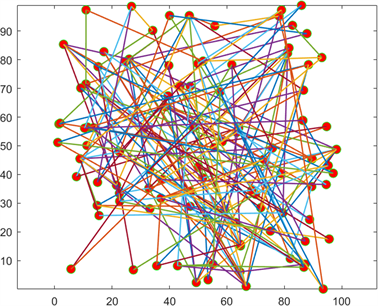Figure 1. Curve: Topology structure of the network (17)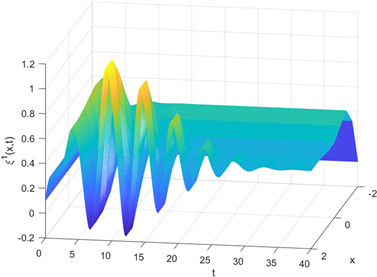Figure 2. Curve: The dynamical behavior of ${\xi }_{}^{1}\left(x,t\right)$Figure 3. Curve: The dynamical behavior of ${\xi }_{}^{2}\left(x,t\right)$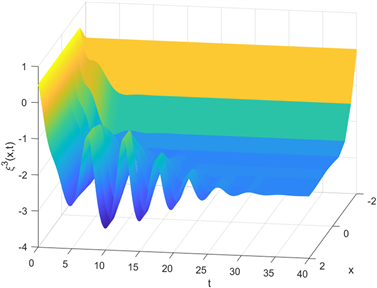Figure 4. Curve: The dynamical behavior of ${\xi }_{}^{3}\left(x,t\right)$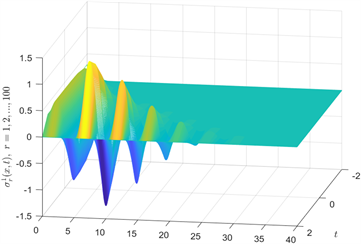Figure 5. Curve: Synchronization error ${\sigma }_{r}^{1}\left(x,t\right)$Figure 6. Curve: Synchronization error ${\sigma }_{r}^{2}\left(x,t\right)$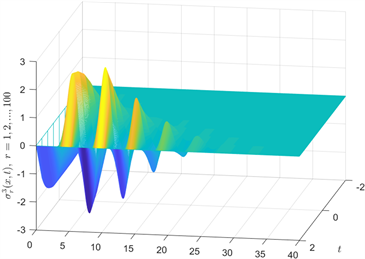Figure 7. Curve: Synchronization error ${\sigma }_{r}^{3}\left(x,t\right)$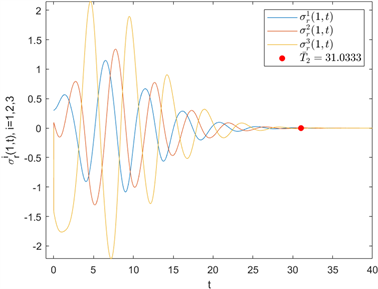Figure 8. Curve: Fixed-time synchronization error of (17)

5. 结论

  Dubey, B., Das, B. and Hussain, J. (2001) A Predator-Prey Interaction Model with Self and Cross-Diffusion. Ecological Model, 141, 67-76. https://doi.org/10.1016/S0304-3800(01)00255-1  Alonso, S., John, K. and Markus, B. (2011) Complex Wave Patterns in an Effective Reaction-Diffusion Model for Chemical Reactions in Microemulsions. Journal of Chemical Physics, 134, 2014102-R. https://doi.org/10.1063/1.3559154  Pecora, L. and Carroll, T. (1999) Master Stability Functions for Synchro-nized Coupled Systems. International Journal of Bifurcation and Chaos, 9, 2315-2320. https://doi.org/10.1142/S0218127499001814  Wei, X., Wu, X.Q, Chen, S.H., Lu, J.A. and Chen, G.R. (2018) Cooperative Epidemic Spreading on a Two-Layered Interconnected Network. SIAM Journal on Applied Dynamical Systems, 17, 1503-1520. https://doi.org/10.1137/17M1134202  Tao, Y. and Chua, L. (1997) Impulsive Stabilization for Control and Synchronization of Chaotic Systems: Theory and Application to Secure Communication. IEEE Transactions on Circuits and Systems: Fundamental Theory and Applications, 44, 976-988. https://doi.org/10.1109/81.633887  Wu, K.N., Tian, T. and Wang, L.M. (2016) Synchronization for a Class of Coupled Linear Partial Differential Systems via Boundary Control. Journal of the Franklin Institute, 353, 4026-4073. https://doi.org/10.1016/j.jfranklin.2016.07.019  He, H. (2020) Asymptotical Synchronization of Coupled Time-Delay Partial Differential Systems via Pinning Control and Boundary Control. International Journal of Advanced Networking and Applications, 11, 4443-4450. https://doi.org/10.35444/IJANA.2020.11062  Yang, C.D., Cao, J.D., Huang, T.W., Zhang, J.B. and Qiu, J. (2018) Guaranteed Cost Boundary Control for Cluster Synchronization of Complex Spatio-Temporal Dynamical Net-works with Community Structure. Science China Information Sciences, 61, Article ID: 052203. https://doi.org/10.1007/s11432-016-9099-x  Bhat, S.P. and Bernstein, D.B. (2000) Finite-Time Stability of Continuous Autonomous Aystems. SIAM Journal on Control and Optimization, 38, 751-766. https://doi.org/10.1137/S0363012997321358  Franceschelli, M., Pisano, A., Giua, A. and Usai, E. (2015) Fi-nite-Time Consensus with Disturbance Rejection by Discontinuous Local Interactions in Directed Graphs. IEEE Transactions on Automatic Control, 60, 1133-1138. https://doi.org/10.1109/TAC.2014.2351431  Hu, Y.A., Li, H.Y., Zhang, C.P. and Liu, L. (2012) Robust Adaptive Finite-Time Synchronization of Two Different Chaotic Systems with Parameter Uncertainties. Journal of Applied Mathematics, 2012, Article ID: 607491. https://doi.org/10.1155/2012/607491  Luo, Y.P., Ling, Z.M. and Yao, Y.J. (2019) Finite Time Synchronization for Reactive Diffusion Complex Networks via Boundary Control. IEEE Access, 7, 68628-68635. https://doi.org/10.1109/ACCESS.2019.2957547  Wu, K.N., Sun, H.X., Peng, S. and Cheng, C.L. (2017) Fi-nite-Time Boundary Stabilization of Reaction Diffusion Systems. International Journal of Robust and Nonlinear Control, 28, 1-12. https://doi.org/10.1002/rnc.3977  Polyakov, A. (2012) Nonlinear Feedback Design for Fixed-Time Stabilization of Linear Control Systems. IEEE Transactions on Automatic Control, 57, 2106-2110. https://doi.org/10.1109/TAC.2011.2179869  Hu, C., He, H.B. and Jiang, H.J. (2020) Fixed/Preassigned-Time Synchronization of Complex Networks via Improving Fixed-Time Stability. IEEE Transactions on Cybernetics, 1-11. https://doi.org/10.1109/TCYB.2020.2977934  Ji, G.J, Hu, C., Yu, J. and Jiang, H.J. (2018) Finite-Time and Fixed-Time Synchronization of Discontinuous Complex Networks: A Unified Control Framework Design. Journal of the Franklin Institute, 355, 4665-4685. https://doi.org/10.1016/j.jfranklin.2018.04.026  Wang, Z.Y., Cao, J.D., Cai, Z.W. and Rutkowski, L. (2019) Anti-Synchronization in Fixed Time for Discontinuous Reaction-Diffusion Neural Networks with Time-Varying Coef-ficients and Time Delay. IEEE Transactions on Cybernetics, 50, 2758-2769. https://doi.org/10.1109/TCYB.2019.2913200  Wang, S.P., Guo, Z.Y., Wen, S.P., Huang, T.W. and Gong, S.Q. (2020) Finite/Fixed-Time Synchronization of Delayed Memristive Reaction-Diffusion Neural Networks. Neurocomputing, 375, 1-8. https://doi.org/10.1016/j.neucom.2019.06.092  Espitia, N., Polyakov, A., Efimov, D. and Perruquetti, W. (2019) Boundary Time-Varying Feedbacks for Fixed-Time Stabilization of Constant-Parameter Reaction-Diffusion Systems. Automatica, 103, 398-407. https://doi.org/10.1016/j.automatica.2019.02.013